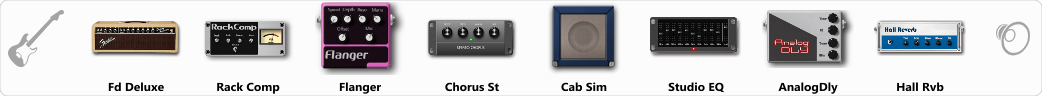Discussion in 'ToneLib-GFX presets' started by Tetragramm1983, Jun 5, 2019.

1. ### Tetragramm1983Active Member

GFX version: 3.0

Optimized for: Guitar Amp

Effects chain:Pickup in Middle Position!

Effect: "Fd Deluxe" (Amp simulators), active - "yes"
"Gain" = 8
"Bass" = 65
"Middle" = 25
"Treble" = 75
"Presence" = 25
"Master" = 89
"Output" = 50
"Level (dB)" = 10

Effect: "Rack Comp" (Dynamics / Filter), active - "yes"
"Threshold (dB)" = -12
"Ratio" = 5
"Attack" = Fast
"Release (ms)" = 250
"Knee" = 4
"Level (dB)" = 14

Effect: "Flanger" (Modulation / Sfx), active - "yes"
"Speed" = 1.9
"Depth" = 100
"Reso" = 35
"Center" = 10.0
"Offset" = 1.4
"Mix" = 61
"Reset" = Off

Effect: "Chorus St" (Modulation / Sfx), active - "yes"
"Speed" = 1.9
"Depth" = 100
"Center" = 8.9
"Mix" = 100

Effect: "Cab Sim" (Cabinets), active - "yes"
"Model" = 4x10" Tweed P10r
"Level (dB)" = 4

Effect: "Studio EQ" (Dynamics / Filter), active - "yes"
"31 Hz" = -12
"62 Hz" = -12
"125 Hz" = 4
"250 Hz" = -10
"500 Hz" = -13
"1 kHz" = -4
"2 kHz" = 0
"4 kHz" = -3
"8 kHz" = -10
"16 kHz" = -15
"above 16 kHz" = 0
"Level (dB)" = 0

Effect: "AnalogDly" (Delay), active - "yes"
"Time" = Note sync, 1/8 Triplet
"Feedback" = 85
"Tone" = 35
"Mix" = 100

Effect: "Hall Rvb" (Reverb), active - "yes"
"Time" = 7.7
"PreDelay" = 5
"LoDamp" = 32
"HiDamp" = 51
"Mix" = 38

Note: This is a ToneLib-GFX preset file, you will need to download and install the ToneLib-GFX software to use the preset.